Home | | Engineering Mathematics II | Vector Calculus

# Vector Calculus

1 Gradient-Directional Derivative 2. Divergence And Curl –Irrotational And Solenoidal Vector Fields Divergence 3 Vector Integration 4 Green’s Theorem In A Plane;(Excluding proof) 5 Gauss Divergence Theorem:(Excluding proof) 6 Stoke’s Theorem(Excluding proof)

VECTOR CALCULUS

2.       Divergence And Curl –Irrotational And Solenoidal Vector Fields Divergence

3        Vector Integration

4        Green’s   Theorem   In   A   Plane;(Excluding proof)

5        Gauss Divergence Theorem:(Excluding proof)

6        Stoke’s   Theorem(Excluding   proof)

VECTOR CALCULUS

1(a) The Vector Differential Operator1(b) The Gradient (Or Slope Of A Scalar Point Function)1.2.  Directional Derivative1.3. Unit Tangent Vector1.Find  a unit tangent vector to the following surfaces at the specified1.4 Normal Derivative1.5 Unit Normal Vector1.6 Angle Between The Suraces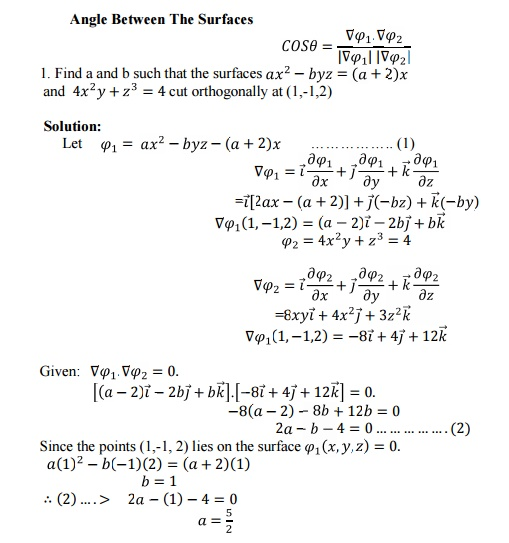1.7 .Scalar Potential1. 8 The Vector Equation of the Tangent Plane And Normal Line to the SurfaceTutorial Problems:2 Divergence And Curl Irotational And Solenoidal Vector Fields:

2.1 Divergence and curl2.2   SOLENOIDAL VECTOR,IRROTATIONAL VECTOR:

Solenoidal vector formula: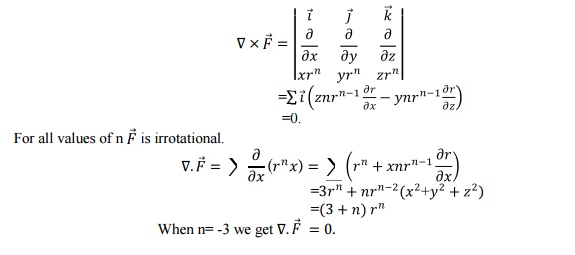Laplace Operator:3 Vector Integration

Conservative Vector Field:3.1. Line Integral:3.2. Surface Integral:

Definition: Consider a surface S .Let n denote the unit outward normal to the surface S. Let R be the projection of the surface x on xy plane. Let Vec f be a vector function defined in some region  containing the surface S, then the surface integral of Vector f is defined to be3.3. Volume Integral: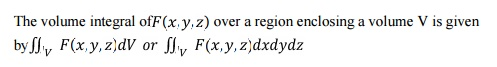3.4 Tutorial Problems: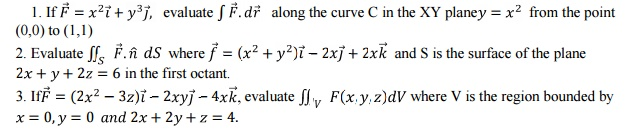4 .Green’s Theorem In A Plane:

Statement:Tutorial Problems:5 Gauss Divergence Theorem:

Statement:

The surface integral of the normal component of a vector function F over a closed surface  S enclosing volume V is equal to the volume integral of the divergence of F taken throughout the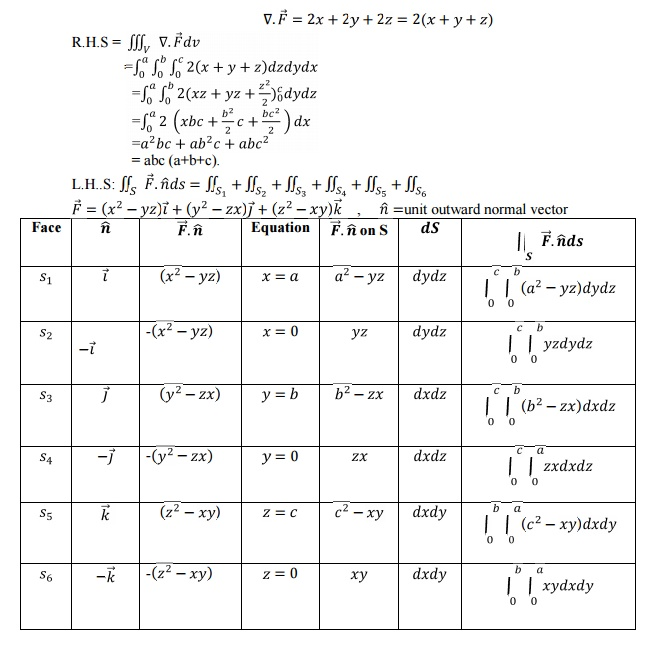6. Stoke’s Theorem

Statement:

The surface integral of the normal component of the curl of a vector function F over an open surface S is equal to the line integral of the tangential component of F around the closed curve C bounding S.Hence, Stoke’s  theorem is verified.

6.1 Tutorial Problems:Study Material, Lecturing Notes, Assignment, Reference, Wiki description explanation, brief detail
Mathematics (maths) : Vector Calculus : Vector Calculus |

Related Topics